The Golden Rectangle and Durer's Spiral

If we start with a golden rectangle we can cut a square and we get a small golden rectangle.From Euclid's definition of the division of a segment into its extreme and mean ratio we introduce a property of golden rectangles and we deduce the equation and the value of the golden ratio.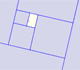A golden rectangle is made of an square and another golden rectangle.

We can repeat this process and we have this construction:Drawing up an arc of circumference in each square we get a golden spiral (also called Durer's Spiral).We have already calculate the polar coordinates of several points and we got a sequence of points.A golden rectangle is made of an square an another golden rectangle. These rectangles are related through an dilative rotation.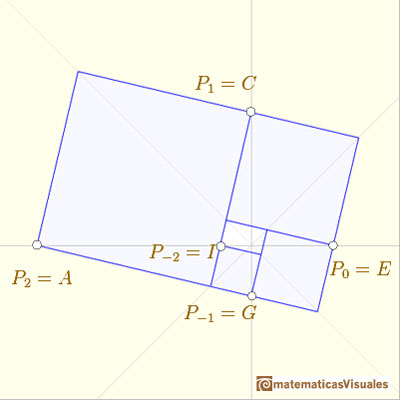These vertices also belong to an equiangular spiralIn an equiangular spiral the angle between the position vector and the tangent is constant.

We are going to calculate the equation of this equiangular spiral.

The general formula for an equiangular spiral is:If n=1 then

We can write for point C

The equation of this equiangular spiral is"This true spiral is closely approximated by the artificial spiral formed by circular quadrants inscribed in the successive squares. (But the true spiral cuts the sides of the squares at very small angles, instead of touching them)" Coxeter.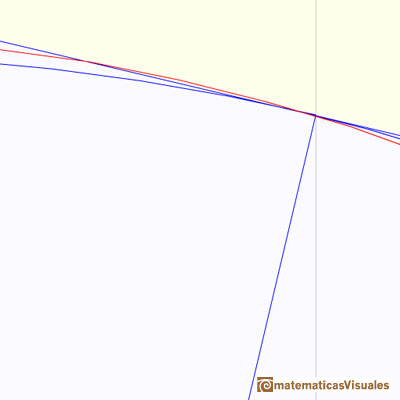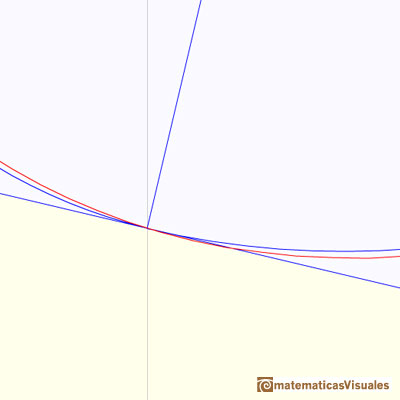In the next applet you can have another look about the relation between the golden rectangle and the dilative rotation. You can see how several points follow the path of an equiangular spiral.

Golden Spiral in a furniture designed and made by Roberto Cardil (pine wood).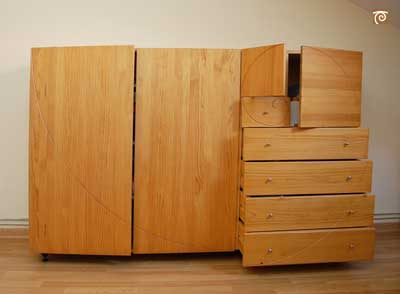REFERENCES

Coxeter H. S. M. - Introduction to Geometry (John Whiley and Sons, Second Edition, 1969)The diagonal of a regular pentagon are in golden ratio to its sides and the point of intersection of two diagonals of a regular pentagon are said to divide each other in the golden ratio or 'in extreme and mean ratio'.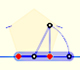You can draw a regular pentagon given one of its sides constructing the golden ratio with ruler and compass.In his book 'Underweysung der Messung' Durer draw a non-regular pentagon with ruler and a fixed compass. It is a simple construction and a very good approximation of a regular pentagon.From Euclid's definition of the division of a segment into its extreme and mean ratio we introduce a property of golden rectangles and we deduce the equation and the value of the golden ratio.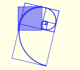The golden spiral is a good approximation of an equiangular spiral.A golden rectangle is made of an square and another golden rectangle.In an equiangular spiral the angle between the position vector and the tangent is constant.Two transformations of an equiangular spiral with the same general efect.A Dilative Rotation is a combination of a rotation an a dilatation from the same point.Some properties of this platonic solid and how it is related to the golden ratio. Constructing dodecahedra using different techniques.The first drawing of a plane net of a regular dodecahedron was published by Dürer in his book 'Underweysung der Messung' ('Four Books of Measurement'), published in 1525 .He studied transformations of images, for example, faces.One eighth of a regular dodecahedon of edge 2 has the same volume as a dodecahedron of edge 1.The twelve vertices of an icosahedron lie in three golden rectangles. Then we can calculate the volume of an icosahedronLeonardo da Vinci made several drawings of polyhedra for Luca Pacioli's book 'De divina proportione'. Here we can see an adaptation of the dodecahedron.